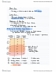# ECO101H1 Study Guide - Midterm Guide: Average Variable Cost, Marginal Revenue, Marginal Cost

111 views4 pages
School
Department
Course
Professorkatrinasavvy and 39529 others unlocked98
Verified Note
98 documents

## Document Summary

Short run (sr) quantities of at least one of the rms resources is xed. Long run (lr) quantities of all of the rms resources can be varied, but its technology is xed. Very long run quantities of all of the rms resources and its technology can be varied economic pro ts = total revenues - opportunity costs. Total product (tp) total output produced for various levels of labour. Marginal product (mp) increase in total product resulting from a one-unit increase in labour slope of the tp curve ( change of tp / change of l ) Average product (ap) total product per unit of labour (tp/l) = total xed cost (independent of the level of output) + total variable cost (increases as output increases) Marginal cost (mc) increase in total cost resulting from a one-unit increase in output slope of the tv curve (change in tc / change in q) Atc=avc (average variable cost) + afc (average xed cost)